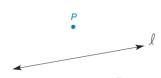Chapter 2.1, Problem 34EElementary Geometry For College St...

7th Edition
Alexander + 2 others
ISBN: 9781337614085

Solutions

Chapter
SectionElementary Geometry For College St...

7th Edition
Alexander + 2 others
ISBN: 9781337614085
Textbook Problem

Given: Line l and point P not on l Construct: P Q ↔ ⊥ lTo determine

To construct:

The perpendicular line on the given line.

Explanation

Given:

Line l and point P not on l.

The given figure is,

Approach:

The given figure is,

Use compass to make an arc from P that intersect l at point C and D as shown in figure (2).

Figure (2)

With C and D as centre mark two arcs equal radii DP to intersect at point Q as shown in figure (3)

Still sussing out bartleby?

Check out a sample textbook solution.

See a sample solution

The Solution to Your Study Problems

Bartleby provides explanations to thousands of textbook problems written by our experts, many with advanced degrees!

Get Started

Perform the indicated operation for the following. 8.

Contemporary Mathematics for Business & Consumers

The graph of θ = 2 in polar coordinates is a: circle line spiral 3-leaved rose

Study Guide for Stewart's Single Variable Calculus: Early Transcendentals, 8th

0 1 π It does not exist.

Study Guide for Stewart's Multivariable Calculus, 8th